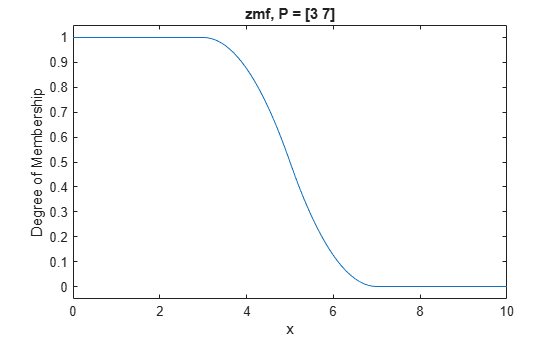# zmf

Z-shaped membership function

## Syntax

``y = zmf(x,params)``

## Description

This function computes fuzzy membership values using a spline-based Z-shaped membership function. You can also compute this membership function using a `fismf` object. For more information, see fismf Object.

This membership function is related to the `smf` and `pimf` membership functions.

example

````y = zmf(x,params)` returns fuzzy membership values computed using the spline-based Z-shaped membership function given by:$f\left(x;a,b\right)=\left\{\begin{array}{ll}1,\hfill & x\le a\hfill \\ 1-2{\left(\frac{x-a}{b-a}\right)}^{2},\hfill & a\le x\le \frac{a+b}{2}\hfill \\ \text{2}{\left(\frac{x-b}{b-a}\right)}^{\text{2}},\hfill & \frac{a+b}{2}\le x\le b\hfill \\ 0\hfill & x\ge b\hfill \end{array}$To define the membership function parameters, specify `params` as the vector [a b].Membership values are computed for each input value in `x`.```

## Examples

collapse all

Specify input values across the universe of discourse.

`x = 0:0.1:10;`

Evaluate membership function for the input values.

`y = zmf(x,[3 7]);`

Plot the membership function.

```plot(x,y) title('zmf, P = [3 7]') xlabel('x') ylabel('Degree of Membership') ylim([-0.05 1.05])```## Input Arguments

collapse all

Input values for which to compute membership values, specified as a scalar or vector.

Membership function parameters, specified as the vector [a b]. Parameter a defines the shoulder of the membership function, and b defines its foot.

## Output Arguments

collapse all

Membership value returned as a scalar or a vector. The dimensions of `y` match the dimensions of `x`. Each element of `y` is the membership value computed for the corresponding element of `x`.

## Alternative Functionality

### `fismf` Object

You can create and evaluate a `fismf` object that implements the `zmf` membership function.

```mf = fismf("zmf",P); Y = evalmf(mf,X);```

Here, `X`, `P`, and `Y` correspond to the `x`, `params`, and `y` arguments of `zmf`, respectively.

## Version History

Introduced before R2006a

expand all